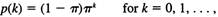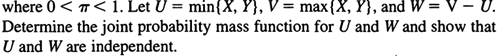### Create an Account

Home / Questions / Let X and Y be independent random variables sharing the geometric distribution whose mass ...

# Let X and Y be independent random variables sharing the geometric distribution whose mass function is

Let X and Y be independent random variables sharing the geometric distribution whose mass function isAug 04 2020 View more View LessSubscribe To Get Solution